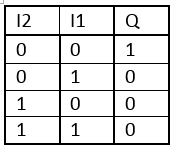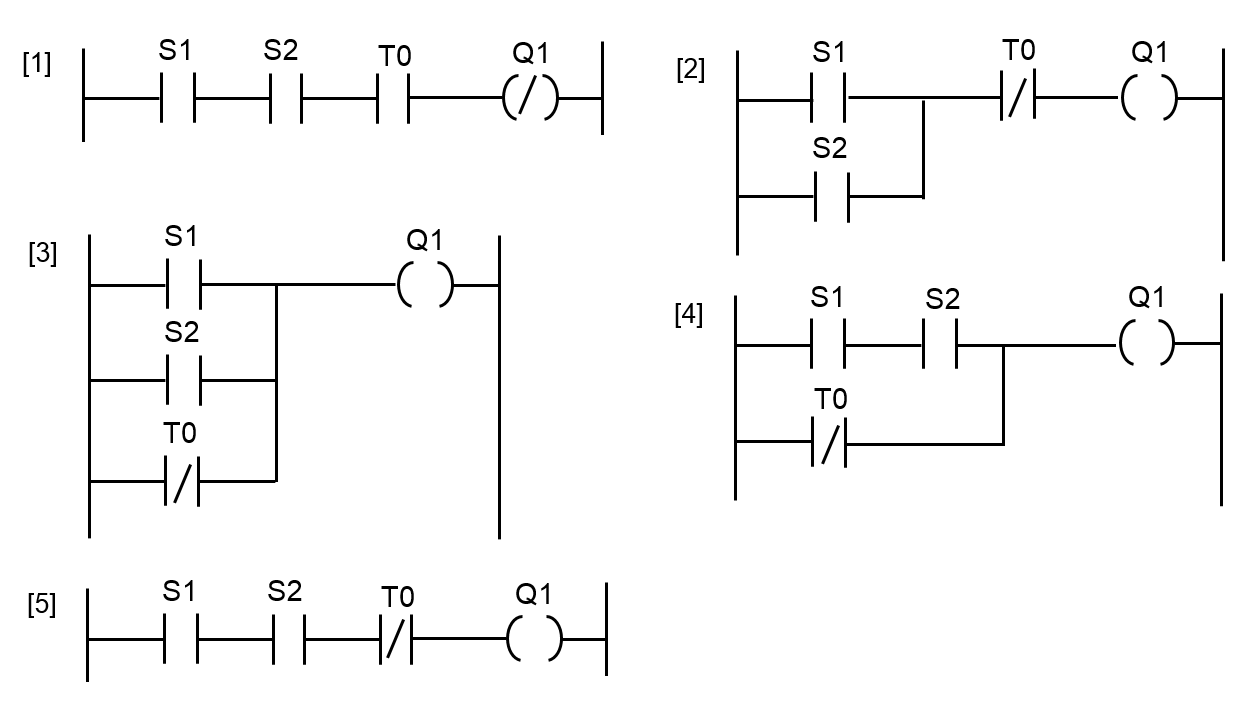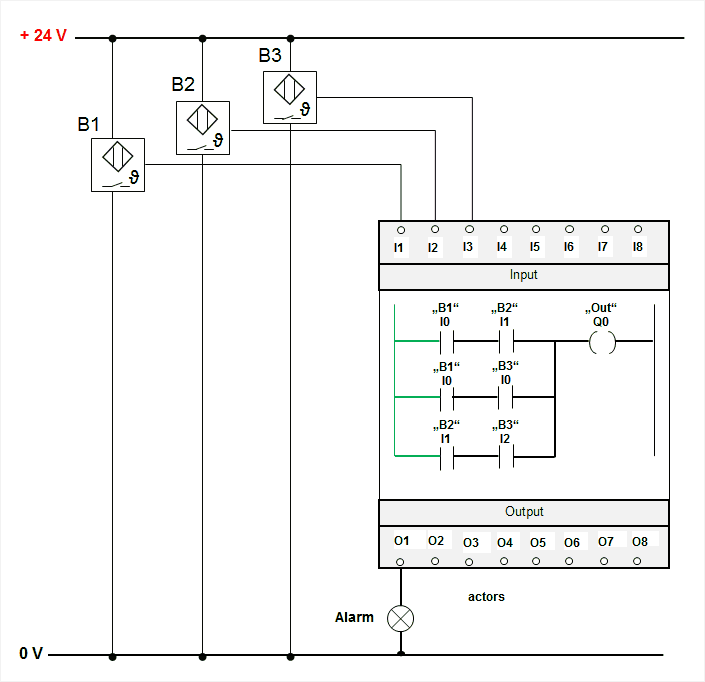(Deutsch)Learnchannel Nachhilfe

### Check Yourself!

The output should only switch to state “1” if only one input has a logical “1” at a time. Which logical function do you have to use here?NOR

Which logical function is represented in this truth table?

A fan should run (Q1 = 1) when the main switch is actuated (S = 1), the temperature sensor (bimetal) reports overtemperature (T = 0) and the motor is running (S2 = 1). Which logical function can be applied to this task?Which statement about the function of this PLC program is correct?PLC – 2 from 3 circuit

Which statement about the following logical function is correct?Interlocking Outputs in PLC